## Introduction

I am continuing to learn `ggplot2` for elegant graphics. I often make a plot to illustrate the fit of a von Bertalanffy growth function to data. In general, I want this plot to have:

• Transparent points to address over-plotting of fish with the same length and age.
• A fitted curve with a confidence polygon over the range of observed ages.
• A fitted curve (without a confidence polygon) over a larger range than the observed ages (this often helps identify problematic fits).

Here I demonstrate how to produce such plots with lengths and ages of Lake Erie Walleye (Sander vitreus) captured during October-November, 2003-2014. These data are available in my `FSAdata` package and formed many of the examples in Chapter 12 of the Age and Growth of Fishes: Principles and Techniques book. My primary interest here is in the `tl` (total length in mm) and `age` variables (see here for more details about the data). I focus on female Walleye from location “1” captured in 2014 in this example.

The workflow below requires understanding the minimum and maximum observed ages.

## Fitting a von Bertalanffy Growth Function

Methods for fitting a von Bertalannfy growth function (VBGF) are detailed in my Introductory Fisheries Analyses with R book and in Chapter 12 of Age and Growth of Fishes: Principles and Techniques book. Briefly, a function for the typical VBGF is constructed with `vbFuns()`1.

Reasonable starting values for the optimization algorithm may be obtained with `vbStarts()`, where the first argument is a formula of the form `lengths~ages` where `lengths` and `ages` are replaced with the actual variable names containing the observed lengths and ages, respectively, and `data=` is set to the data.frame containing those variables.

The `nls()` function is typically used to estimate parameters of the VBGF from the observed data. The first argument is a formula that has `lengths` on the left-hand-side and the VBGF function created above on the right-hand-side. The VBGF function has the `ages` variable as its first argument and then `Linf`, `K`, and `t0` as the remaining arguments (just as they appear here). Again, the data.frame with the observed lengths and ages is given to `data=` and the starting values derived above are given to `start=`.

The parameter estimates are extracted from the saved `nls()` object with `coef()`.

Bootstrapped confidence intervals for the parameter estimates are computed by giving the saved `nls()` object to `Boot()` and giving the saved `Boot()` object to `confint()`.

## Preparing Predicted Values for Plotting

Predicted lengths-at-age from the fitted VBGF is needed to plot the fitted VBGF curve. The `predict()` function may be used to predict mean lengths at ages from the saved `nls()` object.

What is need, however, is the predicted mean lengths at ages for each bootstrap sample, so that bootstrapped confidence intervals for each mean length-at-age can be derived. To do this with `Boot()`, `predict()` needs to be embedded into another function. For example, the function below does the same as `predict()` but is in a form that will work with `Boot()`.

Predicted mean lengths-at-age, with bootstrapped confidence intervals, can then be constructed by giving `Boot()` the saved `nls()` object AND the new prediction function in `f=`. The `Boot()` code will thus compute the predicted mean length at all ages between -1 and 12 in increments of 0.22. I extended the age range outside the observed range of ages as I want to see the shape of the curve nearer t0 and at older ages (to better see L).

The vector of ages, the predicted mean lengths-at-age (from `predict()`), and the associated bootstrapped confidence intervals (from `confint()`) are placed into a data.frame for later use.

For my purposes below, I also want predicted mean lengths only for observed ages. To make the code below cleaner, a new data.frame restricted to the observed ages is made here.

## Constructing the Plot

A `ggplot2` often starts by defining `data=` and `aes()`thetic mappings in `ggplot()`. However, the data and aesthetics should not be set in `ggplot` in this application because information will be drawn from three data.frames – `wf14T`, `preds`, and `preds2`. Thus, the data and aesthetics will be set within specific geoms.

The plot begins with a polygon that encases the lower and upper confidence interval values for mean length at each age. This polygon is constructed with `geom_ribbon()` using `preds2` (the confidence polygon will only cover observed ages) where the x-axis will be `age` and the minimum part of the y-axis will be `LCI` and the maximum part of the y-axis will be `UCI`. The fill color of the polygon is set with `fill=`.3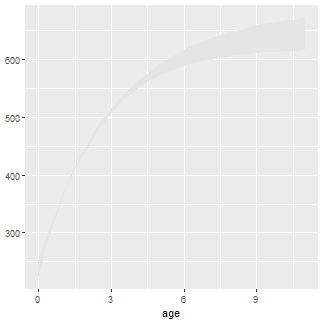Observed lengths and ages in the `wf14T` data.frame were then added to this plot with `geom_point()`. The points are slightly larger than the default (with `size=`) and also with a fairly low transparency value to handle considerable over-plotting.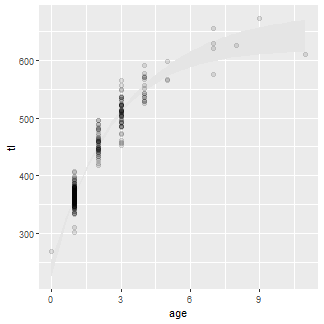The fitted curve over the entire range of ages used above (i.e., using `preds1`) is added with `geom_line()`. A slightly thicker than default (`size=`) dashed (`linetype=`) line was used.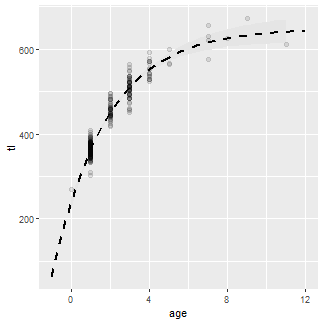The fitted curve for just the observed range of ages (i.e., using `preds2`) is added using a solid line so that the dashed line for the observed ages is covered.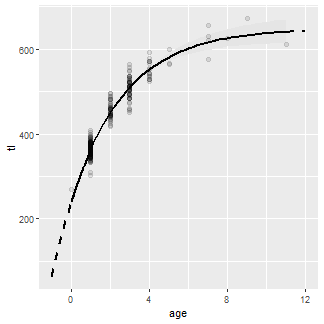The y- and x-axes are labelled (`name=`), expansion factor for the axis limits is removed (`expand=c(0,0)`) so that the point (0,0) is in the corner of the plot, and the axis limits (`limits=`) and breaks (`breaks=`) are controlled using `scale_y_continuous()` and `scale_x_continuous()`.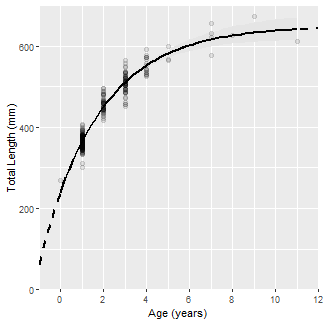Finally, the classic black-and-white theme (primarily to remove the gray background) was used (`theme_bw()` and the grid lines were removed (`panel.grid=`).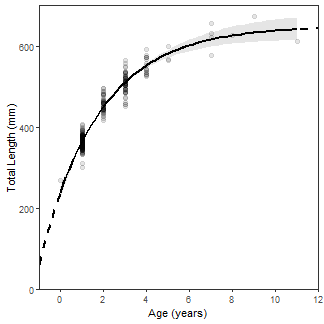## BONUS – Equation on Plot

Below is an undocumented bonus for how to put the equation of the best-fit VBGM on the plot. This is hacky so I would not expect it to be very general (e.g., it likely will not work across facets).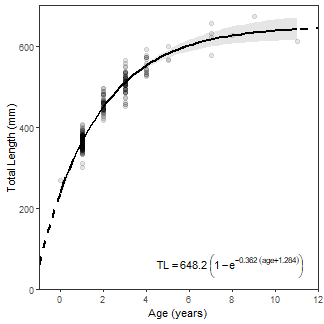## Final Thoughts

This post is likely not news to those of you that are familiar with `ggplot2`. However, I am trying to post some examples here as I learn `ggplot2` in hopes that it will help others. My first post was here. In my next post I will demonstrate how to show von Bertalanffy curves for two or more groups.

## Footnotes

1. Other parameterizations of the VBGF can be used with `param=` in `vbFuns()`. Parameterizations of the Gompertz, Richards, and Logistic growth functions are available in `GompertzFuns()`, `RichardsFuns()`, and `logisticFuns()` of the `FSA` package. See here for documentation. The Schnute four-parameter growth model is available in `Schnute()` and the Schnute-Richards five-parameter growth model is available in `SchnuteRichards()`

2. Reduce the value of `by=` in `seq()` to make for a smoother VBGF curve when plotting later.

3. This polygon will look better in the final plot when the gray background is removed. Also note that the polygon could be outlined by setting `color=` to a color other than what is given in `fill=`